Electroweak Physics at LBL using the CDF detector

In 1979, the Nobel Prize was awarded to Steven Weinberg, Sheldon Glashow and Abdus Salam for the formulation of the theory unifying two of natures forces : the electromagnetic force and the weak nuclear force. At scales significantly smaller than the size of the nucleus the weak nuclear force, which amongst other things underlies the nuclear reactions that fuel the sun, has a strength comparable to the electromagnetic force. The unified electroweak force is mediated by so called gauge bosons : the photon (particles of light), and the Z and W bosons. The experimental discovery of the Z and W bosons in 1983 by the UA1 and UA2 collaborations at CERN was a supreme vindication of the unified electroweak theory and earned Carlo Rubbia and Simon Van der Meer the Nobel Prize in 1984. Since the discovery of the Z and W bosons their properties have been studied with increasing precision. The LEP collider at CERN and the SLC at Stanford have so far produced in excess of 16 million Z bosons, allowing the mass of the Z to be measured with an accuracy of 1 part in 50,000 and confirming that there are no more than three types of neutrino. Although LEP2 has started to run at higher center of mass energies, thereby producing W pairs, the largest sample of W bosons has been produced by the Tevatron collider at Fermilab. Since 1992 some 150,000 W bosons have been detected at the Tevatron.

The CDF group at LBL has and continues to play a leading role in the analysis of W bosons produced at the Tevatron.

The W bosons are produced by quark anti-quark annihilation.The W bosons decay almost immediately, in ~ 10-25 sec, and hence their properties must be measured through their decay products. Experimentally the W bosons are best measured through their decay to a charged lepton (electron or muon) and a neutrino : this decay mode occurs in approximately 22% of W decays. The neutrino is not directly detected and its presence has to be inferred by energy and momentum conservation.

In the pictures below there are three views of a W event produced in the CDF detector.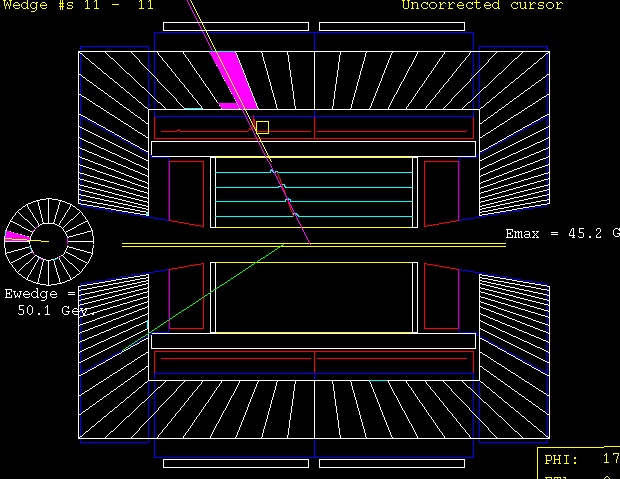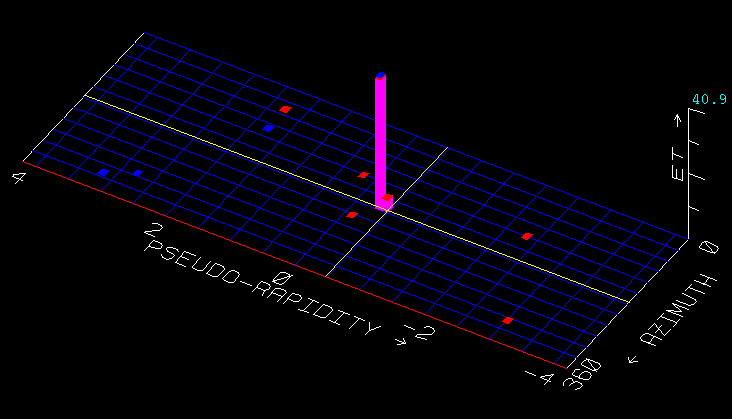The left picture shows the charged tracks produced in the pp collision. The highest momentum track (outlined in the yellow box) is the electron produced from the W decay whilst the red arrow is the inferred neutrino direction (balancing the momentum of the electron). The central picture shows the energy deposited in the calorimeter. The predominant energy deposit is again from the electron produced in the W decay. The final picture shows a side view of the entire CDF detector with the electron track and electron energy deposit from the W decay clearly visible.

 The CDF group at LBL is involved in two measurements of the W boson. A measurement of the W boson mass and a measurement of its decay width.

These measurements in conjunction with other measurements test how adequate our current theoretical description (the so called Standard Model) of elementary particles is. For example the Standard Model predicts the existence of Higgs Bosons (particles responsible for the generation of mass). The mass of the Higgs Boson can be predicted from a precise measurement of the W mass and the top quark mass . A direct measurement of the Higgs boson is one of the major goals of the LHC project currently under construction at CERN.

The current W and top quark mass measurements from the CDF and D0 collaborations are shown below along with the Standard Model predictions for the Higgs Mass.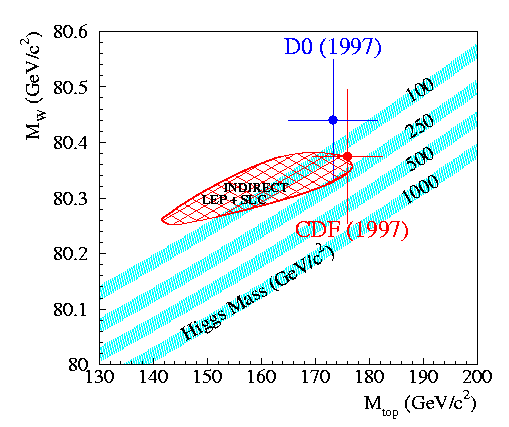At present the constraint on the Higgs mass is rather weak but in the next Tevatron run (run-2) there will be an increase in the data sample by a factor of 20 along with improvements to the CDF detector . This will allow substantially more precise measurements of both the W mass and top quark mass . The two measurements will reduce considerably the range in the predictions for the Higgs Mass.

A measurement of the W width also tests the Standard Model since additional particles beyond the Standard Model would increase the width beyond that predicted by the Standard Model.

The CDF group at LBL has been responsible for a measurement of the W mass using the muon decay channel and a measurement of the W width using the electron decay mode using the so-called run-1B (1994-1995) data set. Approximately 15000 Ws decaying to muons are used in the mass measurement and approximately 50000 Ws decaying to electrons for the width measurement. In both measurements W events are selected by demanding an electron or muon with large transverse momentum (> 25 GeV) in conjunction with the characteristic neutrino signal of missing transverse momentum. The distributions of muon transverse momentum and neutrino transverse momentum (missing ET) are compared to the simulation below.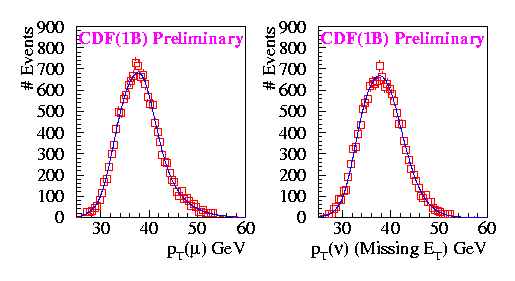The muon and neutrino transverse momentum distributions used in the W mass analysis compared to the simulation.

The track momentum is calibrated by comparing the mass of the J/Psi measured in the CDF tracking detector with the world average value. Approximately 250,000 J/Psis are used in this calibration, allowing a rigorous examination of a large number of systematics which could affect the measurement.A comparison of the 250,000 J/Psi events with the simulation

A sample of approximately 3500 Z events (decaying to both e+e- and µ+µ-) are used as a valuable cross check on the momentum and energy scales and also to fix the parameters which determine the amount of transverse momentum (due to gluon radiation from the quarks) with which the W boson is produced.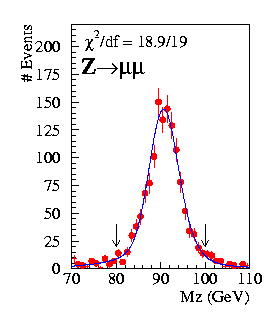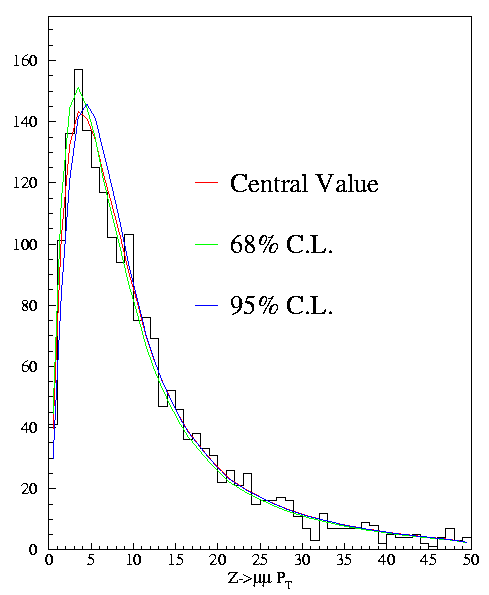(Left) The invariant mass distribution of the Z->µ+µ- events used as a cross check in the momentum scale determination and as a means of determining the resolution of the tracking chamber.
(Right) The transverse momentum distribution of the same Z events. These events are used to constrain the transverse momentum distribution of the W events from which the W mass is measured.

The W mass is measured by comparing the mass reconstructed using the transverse momenta of the charged lepton and neutrino to a detailed simulation. The distribution of this "transverse mass" is shown below for the muon decay channel, from which a W mass of 80.430 +/- 0.100 (stat) +/- 0.135 (syst) GeV was obtained.The transverse mass lineshape of Ws decaying to µ+neutrino, from which the W mass is determined.

The W width is measured by essentially counting the number of events at large values of the transverse mass, since predominantly it is the intrinsic width/lifetime of the W which determines the mass distribution at large values of the mass.The simulated W boson transverse mass distribution for various assumed values of the W width

A preliminary measurement of the W width is shown below. The error is principally dominated by the relatively low number of events (210) in the high transverse mass region (110 < MT < 200 GeV). A width value of 2.19 +/- 0.19 GeV is obtained which is in good agreement with the Standard Model prediction of 2.077 +/- 0.014 GeV. This is currently the most precise, direct measurement of the W width.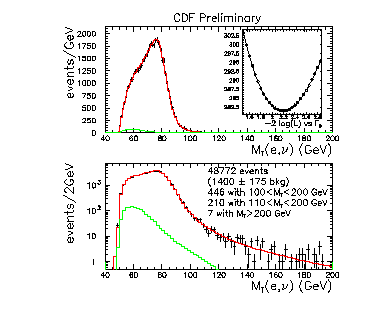The measured W boson (electron decay mode) transverse mass compared to the simulation. The green lines denote the distribution of the background events which are indistinguishable from the true W boson events.

Further information can be found below :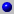Summary of CDF electroweak analysisSummary of the CDF W width analyses

Mark Lancaster